Seventh Mississippi State - UAB Conference on Differential Equations and Computational Simulations. Electron. J. Diff. Eqns., Conference 17 (2009), pp. 185-195.

### Regularity of solutions to doubly nonlinear diffusion equations Jochen Merker

Abstract:
We prove under weak assumptions that solutionsof doubly nonlinear reaction-diffusion equations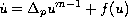to initial values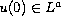are instantly regularized to functions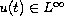(ultracontractivity). Our proof is based on a priori estimates of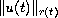for a time-dependent exponent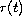. These a priori estimates can be obtained in an elementary way from logarithmic Gagliardo-Nirenberg inequalities by an optimal choice of, and they do not only imply ultracontractivity, but provide further information about the long-time behaviour.

Published April 15, 2009.
Math Subject Classifications: 35K65, 35B35, 46E35, 35B45.
Key Words: p-Laplacian; doubly nonlinear evolution equations; ultracontractive semigroups; logarithmic Gagliardo-Nirenberg inequalities.

Show me the PDF file (219 KB), TEX file, and other files for this article.Jochen Merker University of Rostock, D-18051 Rostock, Germany email: jochen.merker@uni-rostock.de